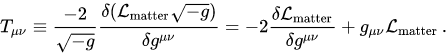# Matter field (Einstein-Hilbert action derivation of the Einstein field equations)

• I
dsaun777
I was going over the Einstein-Hilbert action derivation of the Einstein field equations and came across a term that does not seem to be explicitly defined. That term is the Langragian for the matter fields. What exactly is matter in General relativity in the context of the Lagrangian? Here is one equation with the subscript "matter."•vanhees71

Mentor
In the GR Lagrangian, matter is anything that isn't spacetime geometry; i.e., anything that isn't the Ricci scalar or the metric determinant (or the cosmological constant if that term is included).

•vanhees71
dsaun777
In the GR Lagrangian, matter is anything that isn't spacetime geometry; i.e., anything that isn't the Ricci scalar or the metric determinant (or the cosmological constant if that term is included).
Would that include massless particles?

•vanhees71
Mentor
Would that include massless particles?

As long as they aren't gravitons, yes.

Would that include massless particles?
Yes. Or, for instance, an electromagnetic field. Not mass, but energy-momentum sources gravity.

•vanhees71
Gold Member
2022 Award
As long as they aren't gravitons, yes.
The gravitons would be described by the part of the Lagrangian involving only the metric and its derivatives. In standard GR it's
$$\mathcal{L}=\sqrt{-g} R.$$
This includes also interaction terms of the gravitons, as to be expected from a non-Abelian gauge theory. Gravitons as particles would be defined as the asymptotic free states.

The only trouble is that this doesn't work out as it does for the other interactions, and that's why the quantization of the gravitational field is not yet brought into a satisfactory state :-(.

•dsaun777
Mentor
The gravitons would be described by the part of the Lagrangian involving only the metric and its derivatives.

Yes, that's why I said, in response to the OP, that "matter" includes massless particles other than gravitons, but not gravitons.

•vanhees71
dsaun777
The gravitons would be described by the part of the Lagrangian involving only the metric and its derivatives. In standard GR it's
$$\mathcal{L}=\sqrt{-g} R.$$
This includes also interaction terms of the gravitons, as to be expected from a non-Abelian gauge theory. Gravitons as particles would be defined as the asymptotic free states.

The only trouble is that this doesn't work out as it does for the other interactions, and that's why the quantization of the gravitational field is not yet brought into a satisfactory state :-(.
?
Do you know of any relatively light, no pun intended, material about gravitons?

Gold Member
2022 Award
No. The problem is nobody has found a satisfactory description of gravitons yet.

?
Do you know of any relatively light, no pun intended, material about gravitons?
These notes of Hinterbichler about massive gravity,

https://arxiv.org/abs/1105.3735

are great imo.

•vanhees71
Mentor
massive gravity

Note that, as the introduction of the notes you reference explains, massive gravity is not the same as the standard concept of "gravitons", i.e., massless spin-2 particles.

•vanhees71